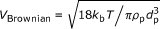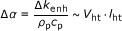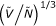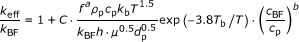## Findings on nanofluid thermal conductivity

A nanofluid  is defined as a mixture of nanosized particles suspended in liquid as the base fluid. The nanofluid is perceived as an extended scope of earlier efforts to study the effective thermal conductivity of multiphase systems containing microscale particle-embedded solid materials  and a solid dispersion in liquid .

Since the first article on measurements of the enhanced thermal conductivity of nanofluids (suspension of Al2O3 and CuO nanoparticles in either water or ethylene glycol) using the transient hot-wire technique was published in 1999 , a number of successive measurement studies have supplemented the original findings and extended the parametric variations affecting the level of conductivity enhancement . These experimental examinations have revealed the parametric importance of thermal conductivity enhancement, including the volume concentration of nanoparticles and their sizes, clustering or aggregation effect, pH effect, surfactant effect, and the base fluid temperature. As a systematic approach, Chon et al.  have constructed an experimentally extrapolated equation that predicts the nanofluid conductivity in terms of the related parameters.

Despite these advances, however, the published studies on theoretical predictions  of the thermal conductivity enhancement of nanofluids continue to be controversial and far from comprehensive. Table 1 shows the chronological presentation of published theories predicting conductivities, either for particle-embedded solid materials or for nanofluids. The first attempt at mathematical modeling dates back to 1873 by Maxwell , who presented effective thermal conductivity for a heterogeneous solid material, consisting of spherical solid particles of thermal conductivity k p embedded in a continuous solid phase with thermal conductivity k BF. The volume concentration f of the embedded spheres is taken to be sufficiently small, such that the spheres do not interact thermally and the effect of the particle size is assumed negligible. In 1962, Hamilton and Crosser  extended Maxwell's model and incorporated a modification for non-spherical particles by the empirical shape factor n.

A number of alternative models have been proposed with the use of the Brownian motion-induced micro-convection in a nanofluid. By adding the second term to the Maxwell model, Xuan et al.  proposed a model incorporating the Brownian motion of nanoparticles in 2003. A year later, Jang and Choi  introduced the Brownian-motion-driven convection model and attempted to describe the temperature-dependency of nanofluid thermal conductivity. They assumed the Nusselt number (Nu) to be the product of Reynolds number (Re) and Prandtl number (Pr), i.e., Nu = Re 2 Pr 2, based on the postulation of Reynolds number of the order of unity. However, this assumption is invalid because it is incorrect to neglect the first two terms, i.e., lower degree terms of Re·Pr, in the expression for the Nusselt number that Acrivos and Taylor  have derived for heat transfer from a spherical particle at low values of the Reynolds number.

Kumar et al.  also attempted to incorporate the nanoparticle thermal conductivity based on the Brownian velocity. However, their model failed as Keblinski et al.  asserted that "the Brownian motion mean free path of a nanoparticle in fluid (by Kumar et al.) is on the order of 1 cm, which is unphysical."

In 2005, Prasher et al.  developed a model combining the Maxwell-Garnett model  incorporating both the Kapitza resistance effect of particles with the surrounding medium and the effect of the Brownian motion-induced convection. Later, they expanded their theoretical prediction for nanofluid thermal conductivity by adding aggregation conductivity contributions for the convection enhancements . However, they assumed a less justifiable Brownian velocity of nanoparticles asbased on the kinetic theory of gas, which is valid just for fine particles suspended in a dilute gas (Boltzmann constant k b = 1.3807 × 10-23J/K, the base fluid temperature T, the nanoparticle densify ρ p, and its diameter d p )--but not quite valid for nanoparticles suspended in liquid. Quite possibly because of this conflict, their model fits only to a subset of experimental data, e.g., agrees fairly well with Al2O3 nanofluid data, but fails to fit to CuO nanofluid data.

The effect of the Brownian motion-induced microconvection remains controversial among different research groups. Eapen et al.  strongly argued that microconvection around randomly moving nanoparticles does not influence the thermal conductivity of the nanofluid. In 2007, Das group proposed a nanofluid thermal conductivity model based on a cell model . Their cell model tried to explain the nonlinear dependence of thermal conductivity of nanofluids on particle volume fraction. However, their empirical constants were defined only to fit to their experimental data. In fact, their model constants did not show consistency for an identical Al2O3 nanofluid.

The kinetic principle well describes the thermal conductivity of gas, as the gas molecules are assumed to be freely moving due to their relatively lean distributions . For liquids, however, their stronger intermolecular forces, primarily because of the higher packing density, make it necessary to modify the kinetic theory. In addition, the molecular collision velocities of gases are too low to explain liquid thermal conductivities that are at least one order of magnitude higher than the gas conductivities. Hence, the thermal conductivities of denser liquids are conjectured to be more properly expressed by the faster sound propagation in the case of liquids, and by the phonon velocity in the case of solids.

In this article, a novel theoretical model describing the nanofluid thermal conductivities, considering all major effective parameters including the size, density and volume concentration of nanoparticles, the fluid temperature and viscosity, and relevant thermal parameters such as thermal conductivity of base fluid and heat capacity of nanoparticles, is proposed and examined for its validity against available experimental data.

## Introduction of heat propagation velocity

The enhanced thermal conductivity of a liquid suspension containing highly conductive metal or metal-oxide particles, such as nanofluids with Au, Al2O3, or CuO, is believed to be attributed to the interaction of nanoparticles with the base fluid molecules. The thermal conductivity of a liquid is given by :(1)

where ρ and c v are the liquid density and specific heat, respectively, u is sonic velocity in liquid, and a is the molecular travel distance between two successive collisions. Likewise, the thermal conductivity enhancement of a nanofluid can include the thermal properties of nanoparticles (ρ p, c p), the heat propagation velocity V ht, which substitutes the sonic velocity, the heat travel distance l ht, which replaces the collision travel distance a, and additional consideration of the volume fraction of nanoparticles f[14, 35]:(2)

Note that the combined term V ht·l ht relates to the increase of thermal diffusivity of nanofluid as.

The heat travel distance l ht, which is defined as the freely traveled distance of heat energy during the interaction of base fluid molecules and nanoparticles, is shown to be equivalent to the root-mean-square displacement of nanoparticles  as:(3)

where μ is the dynamic viscosity of the base fluid and c 1 is a dimensionless proportional constant. In the case of nanofluid, if l ht is assumed to have the same order of magnitude as the mean free path of water molecules, one can estimate l ht ~ 0.170 nm.

The heat propagation velocity can be estimated by examining the order-of-magnitudes of the involved parameters in Equation 2. For example, for 47-nm Al2O3 at 1 vol.% concentration (f·ρ p·c p ~ 3.2 × 104), the thermal conductivity enhancement Δk enh is found to range from 0.025 to 0.100 W/K m . Thus, the heat propagation velocity V ht is estimated to be on the order of 103 m/s. While a more rigorous analysis to determine the heat transfer velocity is yet to be discussed, this estimation is consistent with the conjectures of the characterisitc heat propagation velocity being on the scale of the sound propagation velocity of an order 103 m/s of both in a liquid medium  and in a colloidal medium [37, 38].

The heat propagation velocity V ht represents the heat propagation rate by the vibration of base fluid molecules. In a stationary liquid, individual molecules are constantly moving, and their motions are largely confined within a "cage" formed by the closely packed neighboring molecules . This virtual cage is conceived by the energy barrier of heightwhererepresents the molar free energy of activation for escaping the cage anddenotes the molar Avogadro number. The molecular vibrational frequency ν is given by:(4)

where k b denotes the Boltzmann constant, h and R are the Planck constant and the specific gas constant, respectively, and T is the fluid temperature. The free energy of activation,, is assumed to be constant for a specified fluid and also assumed to be directly related to the internal energy of vaporization at the normal boiling point .

The internal energy is given from Trouton's rule  asandwhereis the enthalpy of vaporization at the normal boiling point T b. Substituting this into Equation 4, and then multiplied by the the heat propagation length scale λ ht , gives an expression for the heat propagation velocity V ht as:(5)

The propagation length scale λ ht , is calculated based on the assumption that the base fluid moledules and nanoparticles are arranged in a cubic lattice, with a center-to-center spacing given by, whereis molar mass of the base fluid.

## New model for nanofluid thermal conductivity

Substituting Equations 3 and 5 into Equation 2 gives an expression for the effective nanofluidic thermal conductivity k eff as:(6)

Two additional modifications of Equation 6 are implemented. First, the volume fraction f is modified to a reduced volume fraction f a (a < 1) to account for the coagulation of nanoparticles that effectively reduce the original volume fraction . The coagulation becomes more severe to require a smaller exponent a with increasing particle concentration because of the decreased inter-particle distance. For example, The surface-to-surface distance of nanoparticles is twice the particle size at 1 vol.%; however, it can decrease to half the particle size at 5 vol.%. Secondly, the effective thermal conductivity of Equation 6 is modified by multiplying the heat capacity ratio of the base fluid to nanoparticles,. It is known that shorter heat dissipation time from nanoparticles into the base fluid enhances the effective thermal conductivity of nanofluid [41, 42]. The heat dissipation time decreases with increasing heat capacity of the base fluid and decreasing heat capacity of the nanoparticles. In other words, nanoparticles with a smaller heat capacity require shorter heat dissipation time to the base fluid, and this results in greater thermal diffusion and higher effective thermal conductivity. The effective conductivity increases in consistency with the heat capacity ratio.

Therefore, after accommodating the above two modifications, the effective thermal conductivity of nanofluids of Equation 6 is given by:(7)

where C is a modified constant and c BF is the base fluid specific heat. The heat transfer length scale λ ht is difficult to be calculated directly, but may be determined by order analysis and merged into the constant C. The exponents a and b are empirical constants that represent the effect of nanoparticle coagulation and of nanoparticle heat dissipation, respectively. A regression analysis of published experimental data by the authors  provides a = 0.70, b = 1.5, and C = 3.58 × 10-14 m for the case of Al2O3 nanoparticles of three different sizes (11 nm, 47 nm, 150 nm diameters) suspended in water under various experimental conditions of a volume concentration range of 1 to 4 vol.% and a tested temperature range of 21 to 71°C.

Figure 1 compares different types of velocity scales that are considered relevant in describing nanofluid thermal conductivity models: (1) three differently defined Brownian velocities for 47-nm Al2O3 nanoparticles , (2) the Brownian velocity of the base fluid (water) molecules , (3) the heat propagation velocity based on the currently proposed model (Equation 5), (4) the sound velocity in water , and (5) phonon velocities for selected solid mediums of αalpha-Fe and silicons [44, 45]. The phonon velocities are expected to be faster than the heat propagation velocity in liquid because of the relatively higher heat conductivities in solid mediums.

Table 2 shows the functional expressions of these velocities and their calculated magnitudes for the tested temperature range of 21 to 71°C. Note that all the previously reported nanofluid thermal conductivity models use the Brownian velocities for the heat propagation velocity, while the present propagation velocity is comparable to the sonic velocity in the base fluid that is several orders larger than the Brownian velocities. The Brownian velocities based on the nanoparticles are too slow to be compatible with the relatively faster heat conduction phenomena in liquids. Furthermore, the Brownian velocities of the base fluid of water molecules are also considered too slow to properly model the nanofluidic conductivities.

Nevertheless, we do not mean that the Brownian motion is not related to the thermal conductivity enhancement. Nor do we mean that Brownian convection is not significant. What we imply is that the assumption in , i.e., the Nusselt number can be expressed as Nu = Re 2 Pr 2, is invalid because it is incorrect to neglect the first two terms, i.e., lower degree terms of Re·Pr, in the expression for the Nusselt number that Acrivos and Taylor  have derived.

In addition, in order to have significant convection effect by wavelength mode of long molecular motion, the bulk fluid needs externally imposed gradients such as pressure, gravity or temperature. However, a nanofluid has quiescent condition, which cannot support any convection [34, 46]. The Brownian velocity, as shown in Figure 1, is several orders of magnitude lower than the required velocity scale of 103 in modeling nanofluid conductivity enhancement.

Figure 2a-c shows the present model for thermal conductivities of water-based nanofluids, Equation 7, in comparison with five published models , for the three different nanofluids. The symbols represent the corresponding experimental data for Al2O3 and CuO [present work]. For all three nanofluids with 47-nm Al2O3 at 1 and 4%, and 30-nm CuO at 1%, Xuan et al.  overestimated the Maxwell's model  for nanofluids. Jang and Choi's model  shows proximity with experimental data for up to about 50°C for 1 vol.% Al2O3 and 40°C for 4 vol.% Al2O3, but substantially deviates thereafter. This deviation beyond a certain temperature is believed to be attributed to their incorrect postulation implied in determining the Nusselt number, as previously noted. For the CuO nanofluid, their model shows large discrepancies throughout the tested temperature range. Additionally, the model by Kumar et al.  wrongly postulates the mean free path of the base fluid, as pointed out by Keblinski et al. , and completely fails to predict nanofuidic thermal conductivities for all presently tested conditions.

Prasher et al. [28, 29] show fairly good agreement with the experiments for the Al2O3 nanofluid, as shown in Figure 2a, c. However, for the CuO nanofluid (Figure 2b), their model underestimates the corresponding experimental data . When completely different model parameters were imposed for CuO from that of Al2O3, the model agrees well with the data; however, the model then lacks comprehensiveness because different model parameters need to be determined for different types of nanofluids. Finally, Patel et al.  agrees fairly well with the experimental data at higher concentrations (Figure 2c) but overestimate the thermal conductivities for low volume concentrations (Figure 2a, b).

In contrast, the present model of Equation 7 shows consistent agreement with the experimental data not only for both nanofluids but for all the tested conditions of temperatures and volume concentrations. Furthermore, Figure 3 demonstrates the comprehensiveness of the present model of Equation 7 in comparison with published experimental data for both Al2O3 and CuO nanofluids from different leading groups [6, 10, 24].